# 详解使用pymysql在python中对mysql的增删改查操作(综合)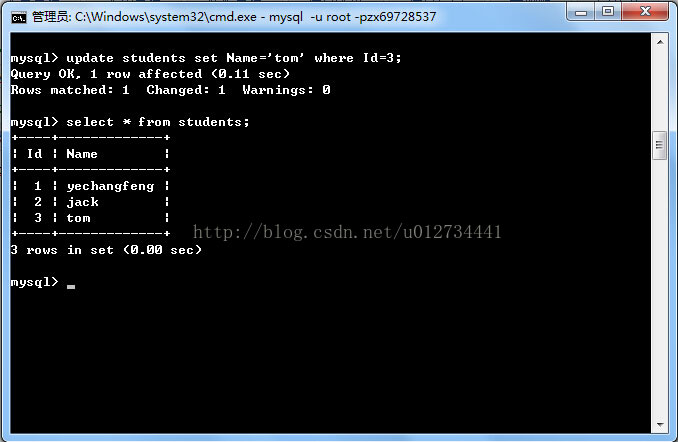```#!/usr/bin/python
#coding:gbk
import pymysql
from builtins import int

#将MysqlHelper的几个函数写出来

def connDB():               #连接数据库
conn=pymysql.connect(host="localhost",user="root",passwd="zx69728537",db="student");
cur=conn.cursor();
return (conn,cur);

def exeUpdate(conn,cur,sql):        #更新或插入操作
sta=cur.execute(sql);
conn.commit();
return (sta);

def exeDelete(conn,cur,IDs):        #删除操作
sta=0;
for eachID in IDs.split(' '):
sta+=cur.execute("delete from students where Id=%d"%(int(eachID)));
conn.commit();
return (sta);

def exeQuery(cur,sql):           #查找操作
cur.execute(sql);
return (cur);

def connClose(conn,cur):          #关闭连接，释放资源
cur.close();
conn.close();

result=True;
print("请选择以上四个操作：1、修改记录，2、增加记录，3、查询记录，4、删除记录.(按q为退出)");
conn,cur=connDB();
number=input();
while(result):
if(number=='q'):
print("结束操作");
break;
elif(int(number)==1):
sql=input("请输入更新语句：");
try:
exeUpdate(conn, cur, sql);
print("更新成功");
except Exception:
print("更新失败");
raise;
elif(int(number)==2):
sql=input("请输入新增语句:");
try:
exeUpdate(conn, cur, sql);
print("新增成功");
except Exception:
print("新增失败");
raise;
elif(int(number)==3):
sql=input("请输入查询语句：");
try:
cur=exeQuery(cur, sql);
for item in cur:
print("Id="+str(item)+" name="+item);
except Exception:
print("查询出错");
raise;
elif(int(number)==4):
Ids=input("请输入Id，并用空格隔开");
try:
exeDelete(conn, cur, Ids);
print("删除成功");
except Exception:
print("删除失败");
raise;
else:
print("非法输入，将结束操作!");
result=False;
break;
print("请选择以上四个操作：1、修改记录，2、增加记录，3、查询记录，4、删除记录.(按q为退出)");
number=input("请选择操作");
```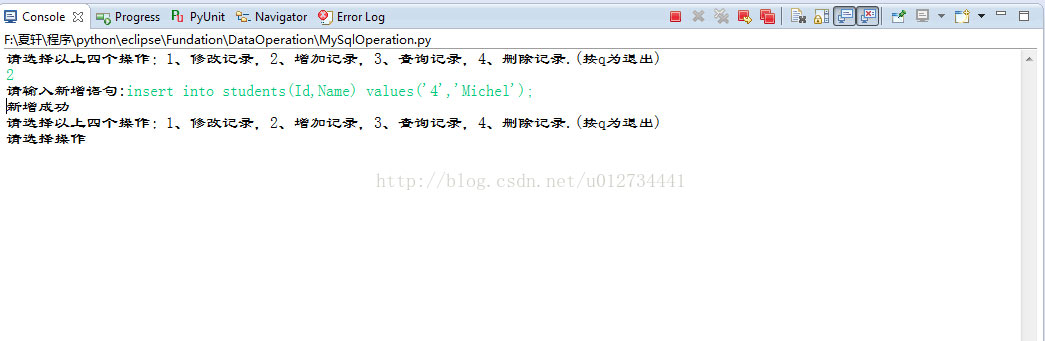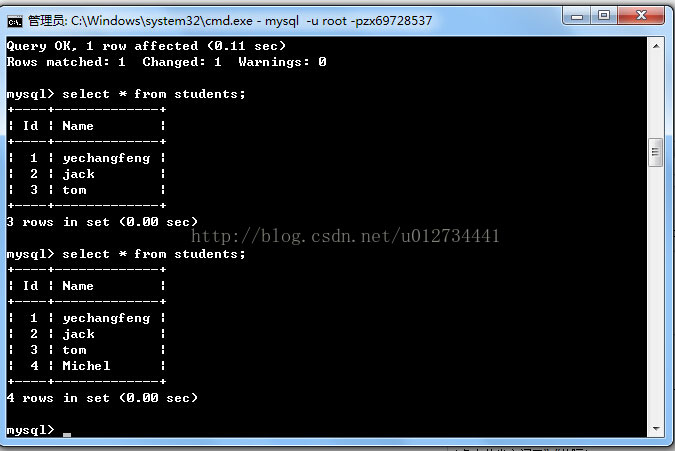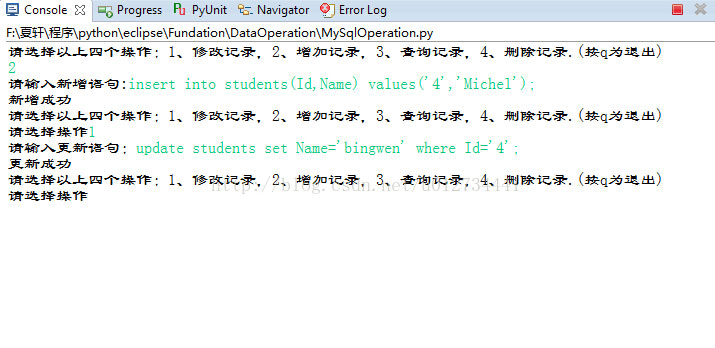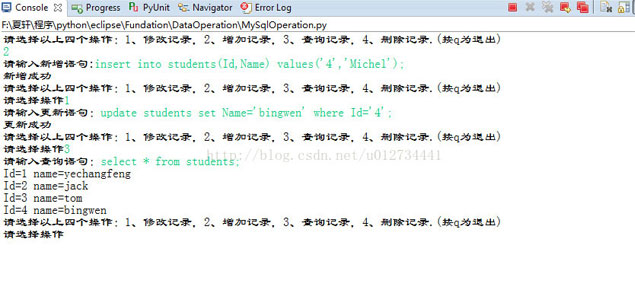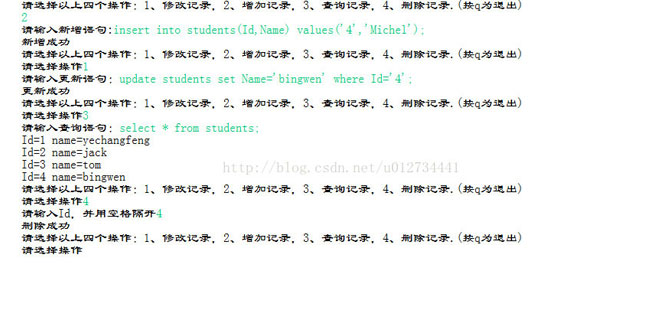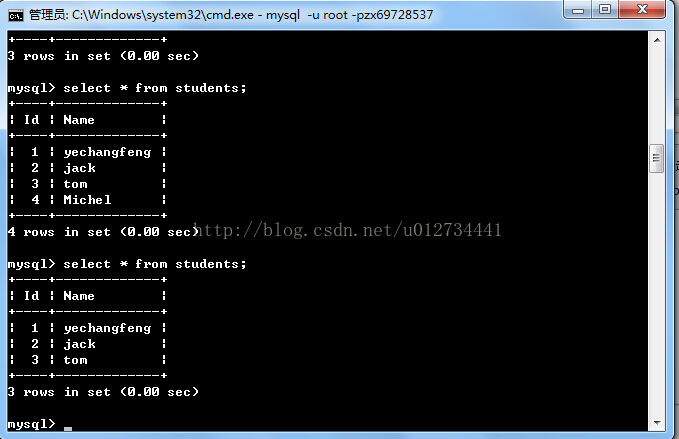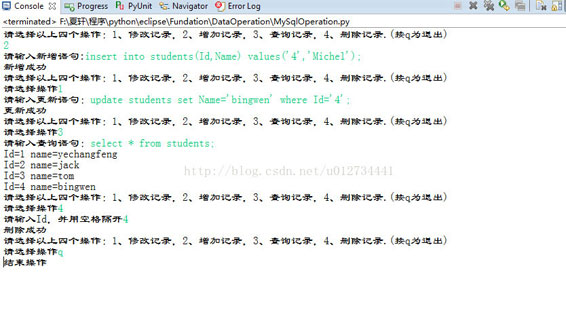python：socket传输大文件示例

Python中的字符串操作和编码Unicode详解